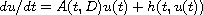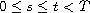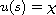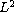Ninth MSU-UAB Conference on Differential Equations and Computational Simulations. Electron. J. Diff. Eqns., Conference 20 (2013), pp. 65-78.

### Modified quasi-reversibility method for nonautonomous semilinear problems Matthew A. Fury

Abstract:
We prove regularization for the ill-posed, semilinear evolution problem,, with initial conditionin a Hilbert space where D is a positive, self-adjoint operator in the space. As in recent literature focusing on linear equations, regularization is established by approximating a solution u(t) of the problem by the solution of an approximate well-posed problem. The approximate problem will be defined by one specific approximation of the operator A(t,D) which extends a recently introduced, modified quasi-reversibility method by Boussetila and Rebbani. Finally, we demonstrate our theory with applications to a wide class of nonlinear partial differential equations inspaces including the nonlinear backward heat equation with a time-dependent diffusion coefficient.

Published October 31, 2013.
Math Subject Classifications: 46C05, 47D06.
Key Words: Regularization for ill-posed problems; semilinear evolution equation; backward heat equation.

Show me the PDF file (261 K), TEX file, and other files for this article.Matthew Fury Division of Science & Engineering, Penn State Abington 1600 Woodland Road Abington, PA 19001, USA email: maf44@psu.edu, Tel: 215-881-7553, Fax: 215-881-7333